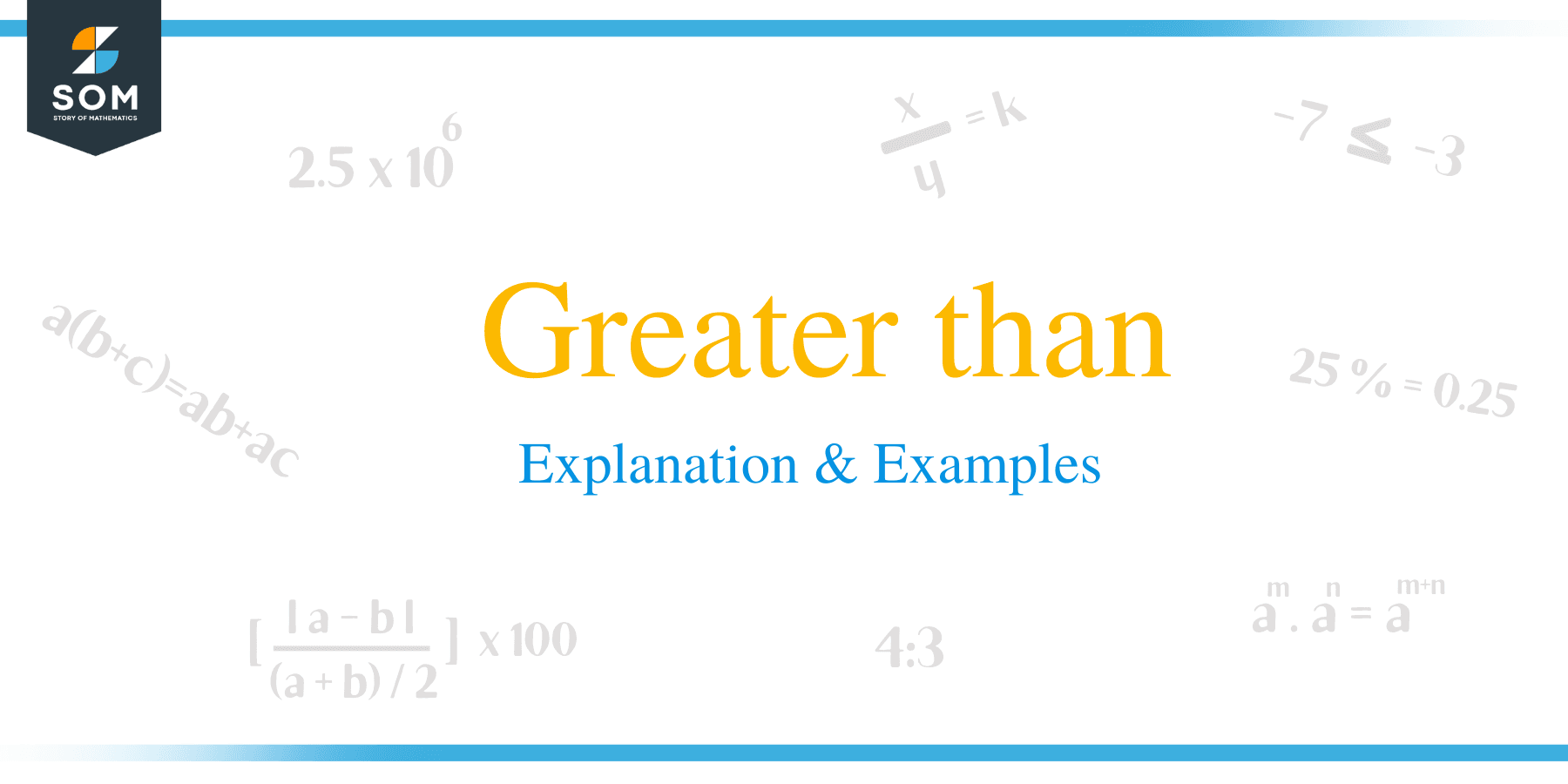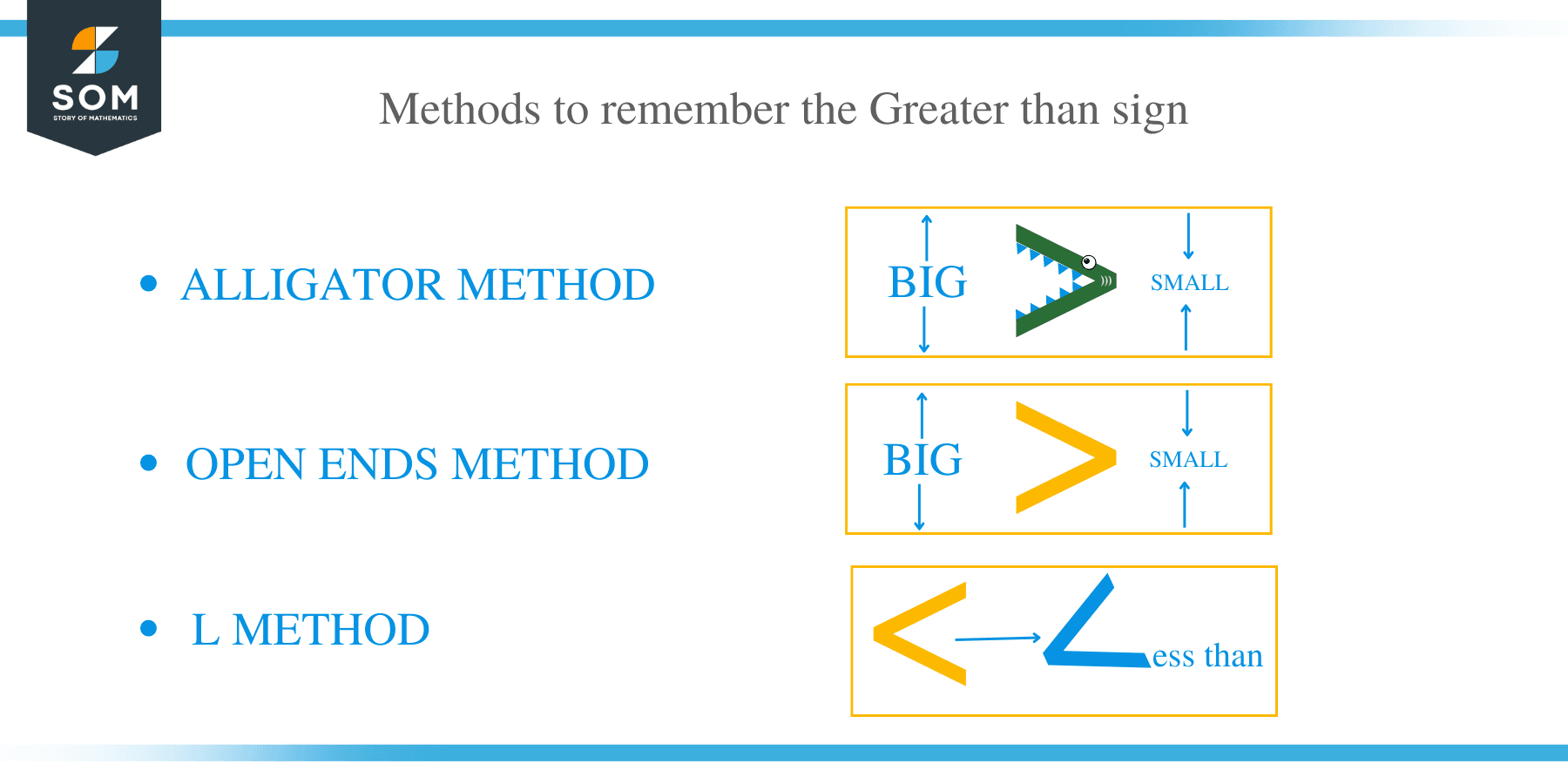# Greater than – Explanation & Examples

##What is Greater Than Sign?

The greater than sign is a mathematical symbol used to denote an inequality between two variables or quantities. This sign has been in use since the 1560s. The sign normally resembles equal – length strokes connecting in acute angle (>).

The symbol is usually placed between two quantities being compared, and it normally shows that the first variable is bigger than the second variable. The greater than sign has been used in computer programming languages to perform other operations

For example, 2 > 1 and 1 > −2. This indicates that 2 is greater than 1 and 1 is greater than negative two.

Some of the examples greater than sign are:

5 > 2: This inequality shows that 5 is greater than 2

45 > 30: 45 is greater than 30

10/2 > 6/3: We can simplify this inequality as 5 > 2: which implies that 5 is greater than 2

0.01 > 0.001 implies that 0.01 is greater than 0.001

2 > -2: In this case, it obvious that positive numbers are greater than negative numbers. Therefore 2 is greater than – 2.## How to Remember Greater than Sign?

There are 3 methods to remember the Greater than sign.

### The alligator method of remembering greater than symbol

The alligator method is the simplest technique for remembering the greater than symbol. Always remind yourself of the alligator when comparing variables using the greater than symbol. The alligator’s mouth is always wide open to swallow or gulp as much food as possible. The mouth of the alligator usually opens to the left.

### The open ends method of remembering greater than symbol

Another easy way to recall the greater than is to remember that open ends of the sign normally face the bigger number, and the arrow points to the smaller number.

### L Method

In this method, recall that the less than starts with the letter L resembles the less than symbol, whereas the greater than symbol does not resemble and sign, therefore because the greater than sign does not look like an L, it cannot there be “less than.”

##Solving Greater than Problems

Before attempting to solve any problem pertaining greater than symbol, the following considerations are made:

• Go through the entire question to understand it.
• Highlight the keywords to help in solving the problem
• Identify the variables
• Write the mathematical expression of the problem using the inequality symbol.
• Justify the expression

Example 1

Saleh has $500 in his savings account at the end of the year. He intends to use at least 200 USD in the account by the start of the following year. If he makes a weekly withdrawal of$25, write an expression describing this situation.

Solution

Start by identifying important keywords.

Assume the variables and let w represent the number of weeks

Therefore, the representation of this situation is:

500 – 25w ≥ 200

In this situation, the greater than or equal sign has been used to cater to the amount to be spent should amount to 200 USD.

Example 2

Brian has fifteen oranges, while Philip has nineteen oranges. Find out the person having more oranges.

Solution

Given,

Brian has 15 oranges.

Philip has 19 oranges.

Since 19 is greater than 15, then we write the inequality as19 >15

Therefore, Philip is having more oranges than Brian.

Example 3

A student cut a 20 m rope into two pieces. How is the shorter and longer piece?

Solution

Let the length of the shorter and longer piece be y and x respectively.

S and L must be more than zero meters and their summation should be equal to 20 m.

Write down all the inequalities:

1. X > 0
2. y > 0
3. x < 20
4. y < 20
5. 0 < x < 20
6. 0 < y < 20
7. y < x

We now combine the expression:

0 < y < x < 20

x + y = 20 m

These inequalities imply that the shorter length y is more than zero, and the longer length x is more than y, while the longer length is less than the total 20 m. Similarly, the sum of the shorter length y and the longer length x is equivalent to 20 m.

### Practice Questions

1. Jake has $\$600$in his savings account at the end of the year. He plans to use at least$\$400$ from his account to purchase holiday presents. If he makes a weekly withdrawal of $\$60$, which of the following expression describes the situation? 2. True or False: Jean baked eighteen cupcakes, while Henry baked sixteen cupcakes. Henry has baked more cupcakes than Jean. 3. True or False: Liz has received eleven gifts, while Jessica received thirteen gifts. Liz has received more gifts than Jessica. 4. True or False: Jacob read eight books over the summer break while Drake read twelves books. This means that Jacob read more books. 5. Sheila is planning to complete DIY woodwork during her holiday break. She cuts a 12 m wood into two pieces:$m$and$n\$ meters. Which of the following best describes the situation?